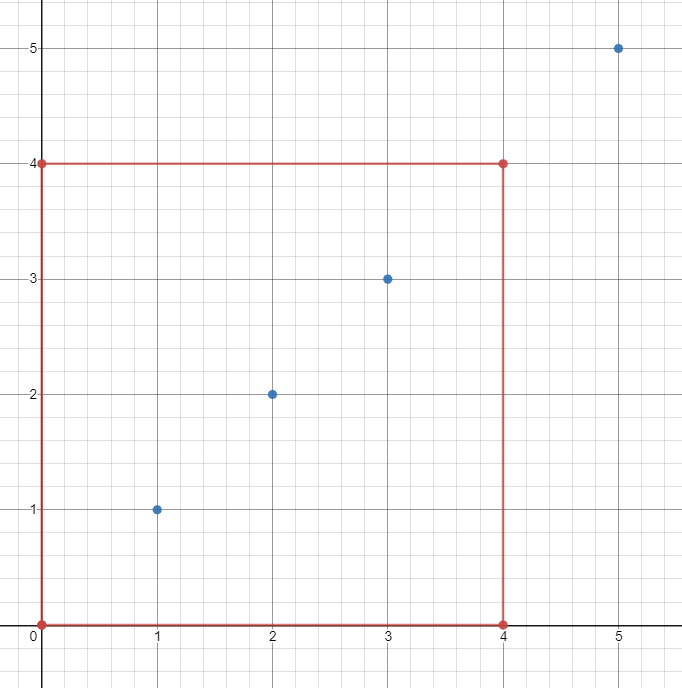1274. Number of Ships in a Rectangle

(This problem is an interactive problem.)

On the sea represented by a cartesian plane, each ship is located at an integer point, and each integer point may contain at most 1 ship.

You have a function `Sea.hasShips(topRight, bottomLeft)` which takes two points as arguments and returns `true` if and only if there is at least one ship in the rectangle represented by the two points, including on the boundary.

Given two points, which are the top right and bottom left corners of a rectangle, return the number of ships present in that rectangle.  It is guaranteed that there are at most 10 ships in that rectangle.

Submissions making more than 400 calls to `hasShips` will be judged Wrong Answer.  Also, any solutions that attempt to circumvent the judge will result in disqualification.

Example :```Input:
ships = [[1,1],[2,2],[3,3],[5,5]], topRight = [4,4], bottomLeft = [0,0]
Output: 3
Explanation: From [0,0] to [4,4] we can count 3 ships within the range.
```

Constraints:

• On the input `ships` is only given to initialize the map internally. You must solve this problem "blindfolded". In other words, you must find the answer using the given `hasShips` API, without knowing the `ships` position.
• `0 <= bottomLeft <= topRight <= 1000`
• `0 <= bottomLeft <= topRight <= 1000`

1274. Number of Ships in a Rectangle
``````struct Solution;

struct Sea {
ships: Vec<Vec<i32>>,
}

impl Sea {
fn new(ships: Vec<Vec<i32>>) -> Self {
Sea { ships }
}

#[allow(non_snake_case)]
fn hasShips(&self, top_right: Vec<i32>, bottom_left: Vec<i32>) -> bool {
for ship in &self.ships {
if ship.inside(&top_right, &bottom_left) {
return true;
}
}
false
}
}

trait Inside {
fn inside(&self, top_right: &[i32], bottom_left: &[i32]) -> bool;
}

impl Inside for Vec<i32> {
fn inside(&self, top_right: &[i32], bottom_left: &[i32]) -> bool {
let x = self;
let y = self;
let x1 = bottom_left;
let y1 = bottom_left;
let x2 = top_right;
let y2 = top_right;
x1 <= x && x <= x2 && y1 <= y && y <= y2
}
}

impl Solution {
fn count_ships(sea: &Sea, top_right: Vec<i32>, bottom_left: Vec<i32>) -> i32 {
if !sea.hasShips(top_right.clone(), bottom_left.clone()) {
0
} else {
let x1 = bottom_left;
let y1 = bottom_left;
let x2 = top_right;
let y2 = top_right;
let x = (x1 + x2) / 2;
let y = (y1 + y2) / 2;
if x1 == x2 && y1 == y2 {
return 1;
}
if x1 == x2 {
let top = Self::count_ships(sea, vec![x1, y2], vec![x1, y + 1]);
let bottom = Self::count_ships(sea, vec![x1, y1], vec![x1, y]);
}
if y1 == y2 {
let left = Self::count_ships(sea, vec![x, y1], vec![x1, y1]);
let right = Self::count_ships(sea, vec![x2, y1], vec![x + 1, y1]);
return left + right;
}
let a = Self::count_ships(sea, vec![x2, y2], vec![x + 1, y + 1]);
let b = Self::count_ships(sea, vec![x, y2], vec![x1, y + 1]);
let c = Self::count_ships(sea, vec![x, y], vec![x1, y1]);
let d = Self::count_ships(sea, vec![x2, y], vec![x + 1, y1]);
a + b + c + d
}
}
}

#[test]
fn test() {
let ships = vec_vec_i32![[1, 1], [2, 2], [3, 3], [5, 5]];
let top_right = vec![4, 4];
let bottom_left = vec![0, 0];
let sea = Sea::new(ships);
let res = 3;
assert_eq!(Solution::count_ships(&sea, top_right, bottom_left), res);
}
``````